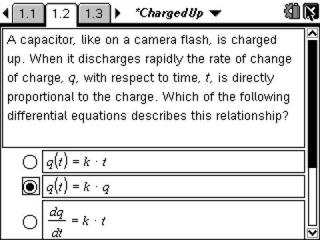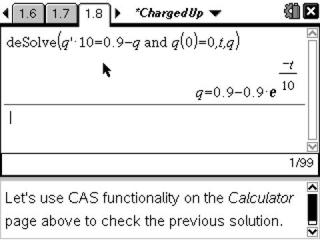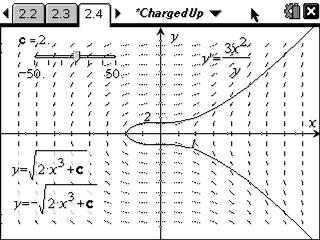# Activities

••• ##### Subject Area

• Math: Calculus: Slope Fields Analysis
• Math: Calculus: Applications of Integral, Antiderivatives and Definite Integrals
• Math: Calculus: Antiderivatives and Slope Fields

• ##### Author9-12

20 Minutes

• ##### Device
• TI-Nspire™ CAS
• ##### Software

TI-Nspire™ CAS

2.0

## Charged Up#### Activity Overview

Use the deSolve command to find general and particular solutions to differential equations. Then dynamically explore the family of particular solution to a differential equation.

#### Key Steps

•Differential Equations are applicable for all sorts of physical phenomena, including radioactive decay, economics, biology, chemistry, population growth and electric circuits with capacitors. Students are asked some exam-like multiple-choice questions to introduce separable differential equations.

•Students use deSolve to find general and particular solutions to differential equations.

•They will also dynamically explore the family of particular solution to a differential equation.